## VA Report Example: Month Average versus Total Average

Started ‎04-04-2018 by
Modified ‎04-04-2018 by
Views 3,737

A report requirement came my way asking for assistance in being able to select a month, calculate that month's average and compare it to the total average for the available data (3 years).

Let's look at the final result and then I will outline how to accomplish this. In this example report, you can see the user selects the month, then that month's Order Total Average is graphed with the full data source's Order Total Average.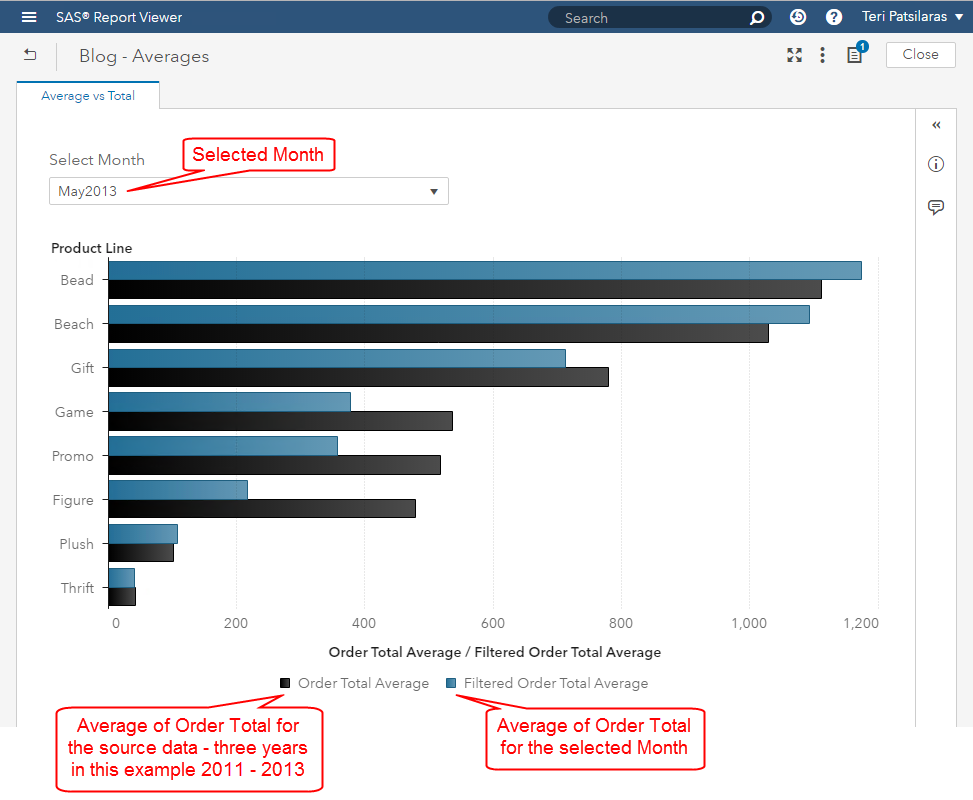Here are the steps to recreate:

Create a selected month parameter with control object

First let's create the parameter which will store the month selection. From the Data pane, select the + New data item and then select Parameter…. Fill out the prompts to best match your data. The Current value is the default value that the report will use when it is opened.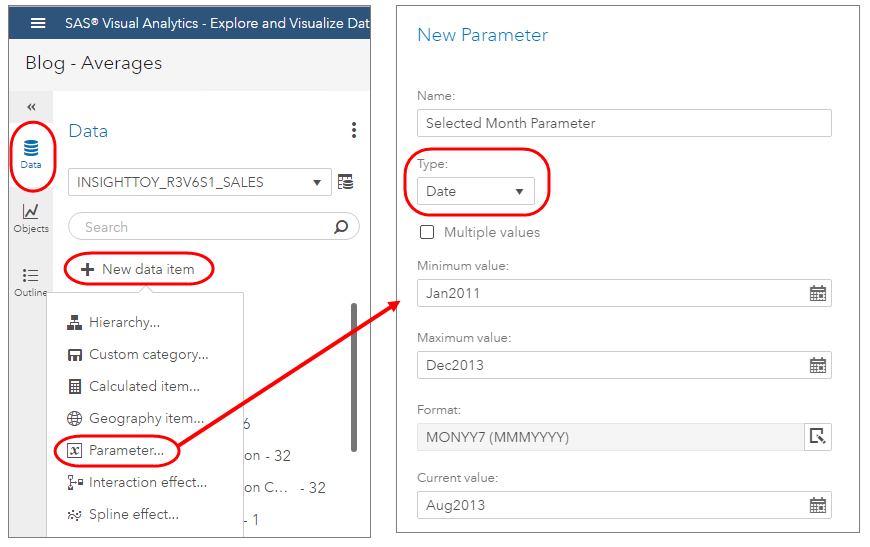Next, we will need to add a control object to the report and assign the parameter role. In this example, I use a Drop-Down List. Be sure to assign the Parameter Role to the newly created parameter above. (Click on the image to enlarge)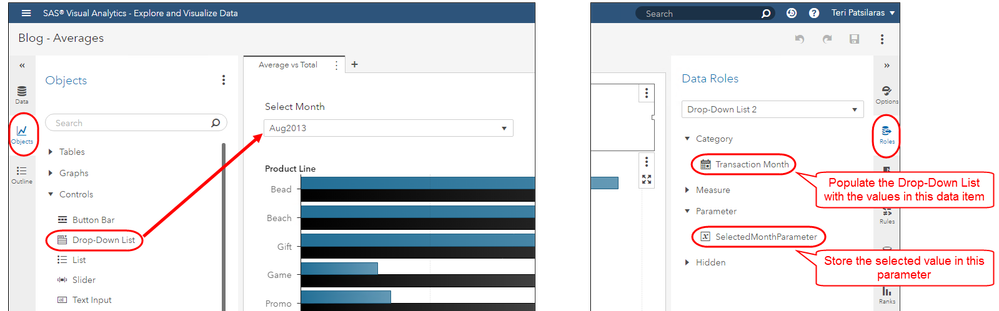# Create the Order Total Average for the available data

Next we will create the average that represents all of the available data. In our example, we have three years' worth of data and we want to use this as the baseline comparison metric.

To do this, we will simply duplicate our measure and then change the aggregation type. Right-click on the target measure and select Duplicate. Then rename the data item and select Average as the Aggregation.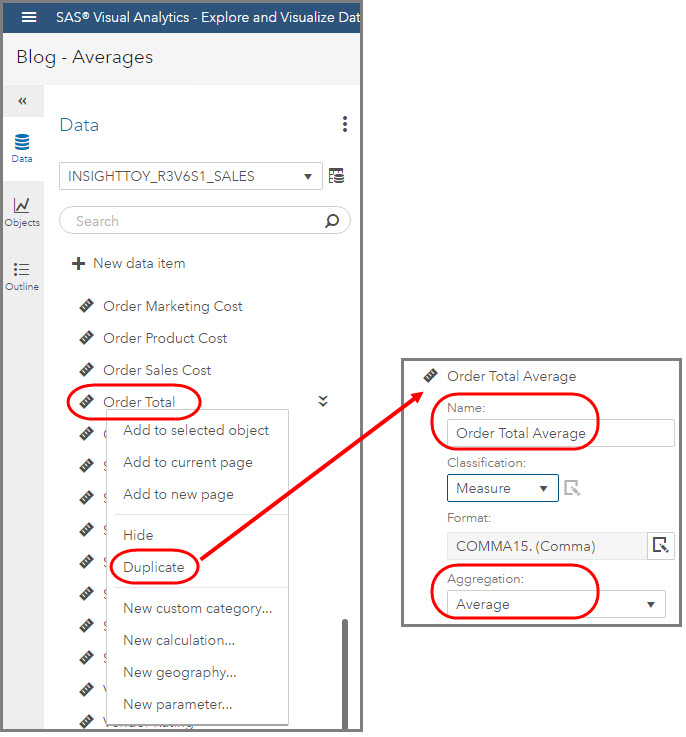Create the Filtered Order Total Average for the selected month

In order to create this measure we will need to create two additional measures to build a compound expression. So in the end, we will have created three new data items to support this requirement. We will need to first create a data item for the Filtered Order Total Sum, then a data item for the Filtered Frequency, and finally we can calculate the ratio for Filtered Order Total Average which equals the Filtered Order Total Sum divided by the Filtered Frequency.

## Filtered Order Total Sum

Use the Data pane's + New data item menu and select Calculated item.... In this expression, we want to only "keep" the values whose Transaction Month equals the selected month from the drop-down list, which is stored in the parameter SelectedMonthParameter. We do not need to do anything explicitly to define the Sum as the default aggregation is SUM.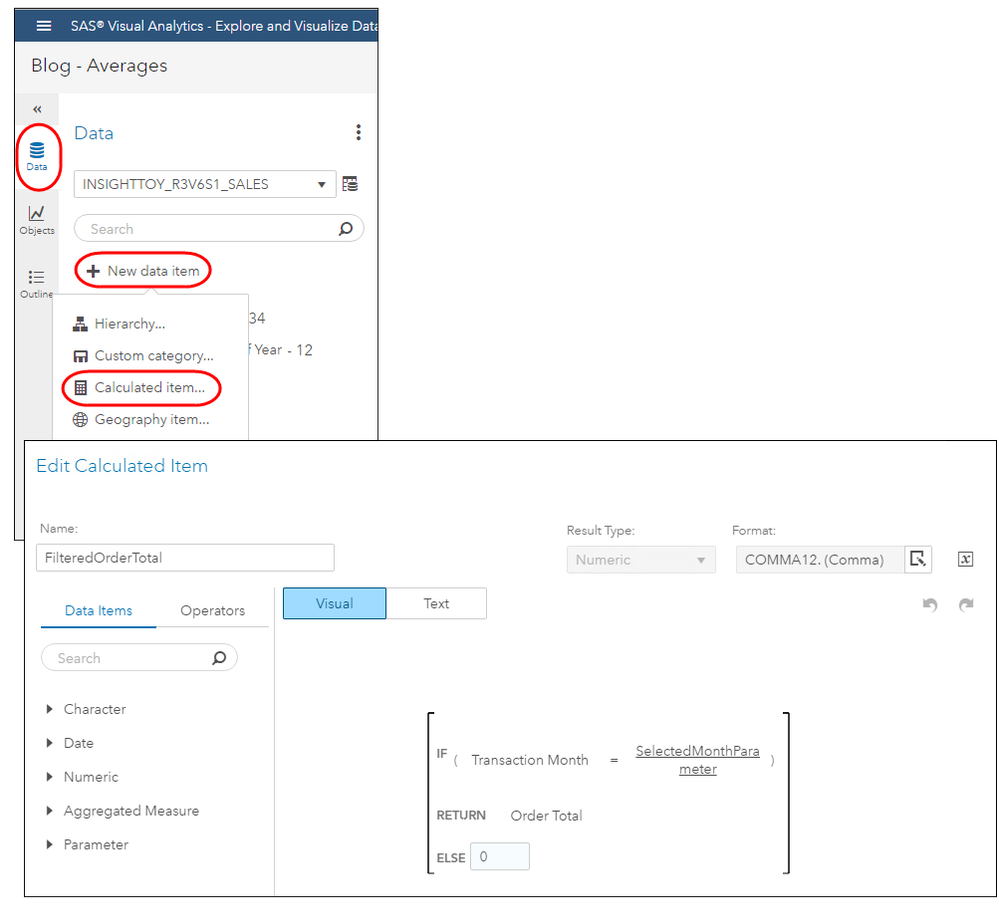Filtered Frequency

Next, we will need to calculate the number of rows whose Transaction Month equals the selected month from the drop-down list, which is stored in the parameter SelectedMonthParameter. Like in the previous step, use the Data pane's + New data item menu and select Calculated item.... Return the Frequency when the expression evaluates true. This will, by default, sum up the number of instances where month equals the selected month.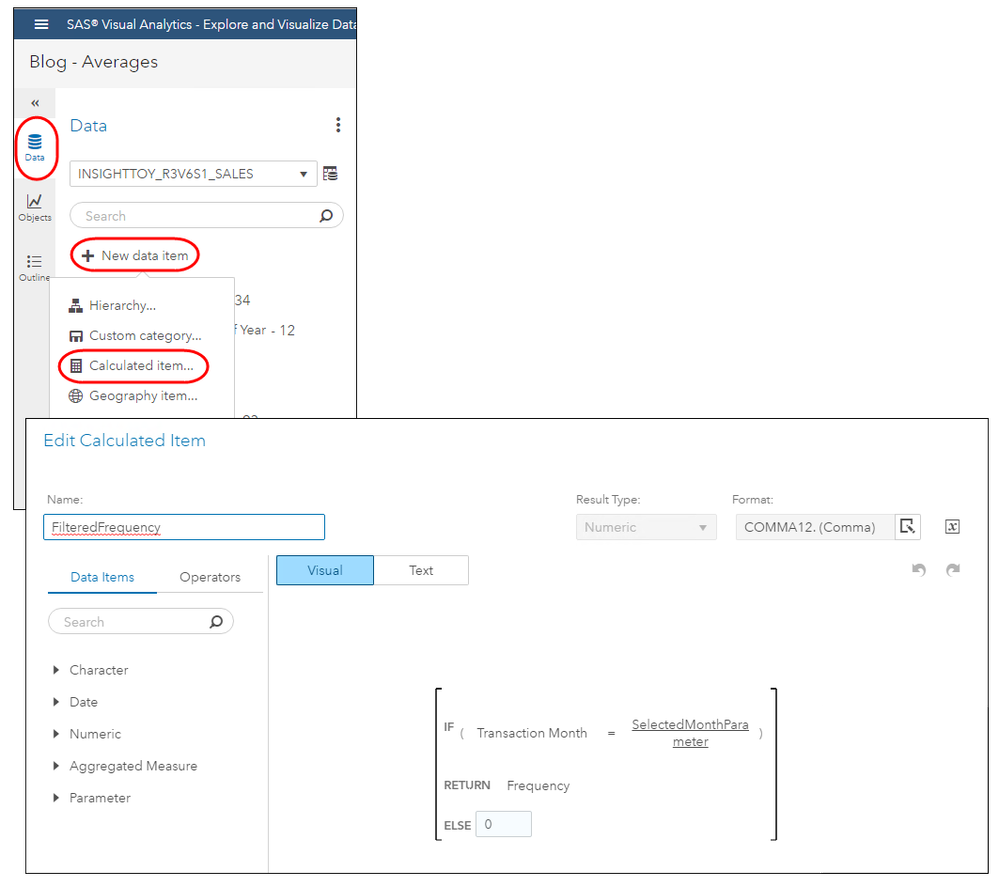## Filtered Order Total Average

Now we can use our two calculated data items to define the expression for the Filtered Order Total Average. This time, when in the expression editor, be sure to select an Aggregated Measure Result Type!

Still use the Data pane's + New data item menu and select Calculated item.... For the Result Type select Aggregated Measure and then define the expression as below. Notice that you will need to use the SUM aggregation operator in your ratio expression. (Click on the image to enlarge)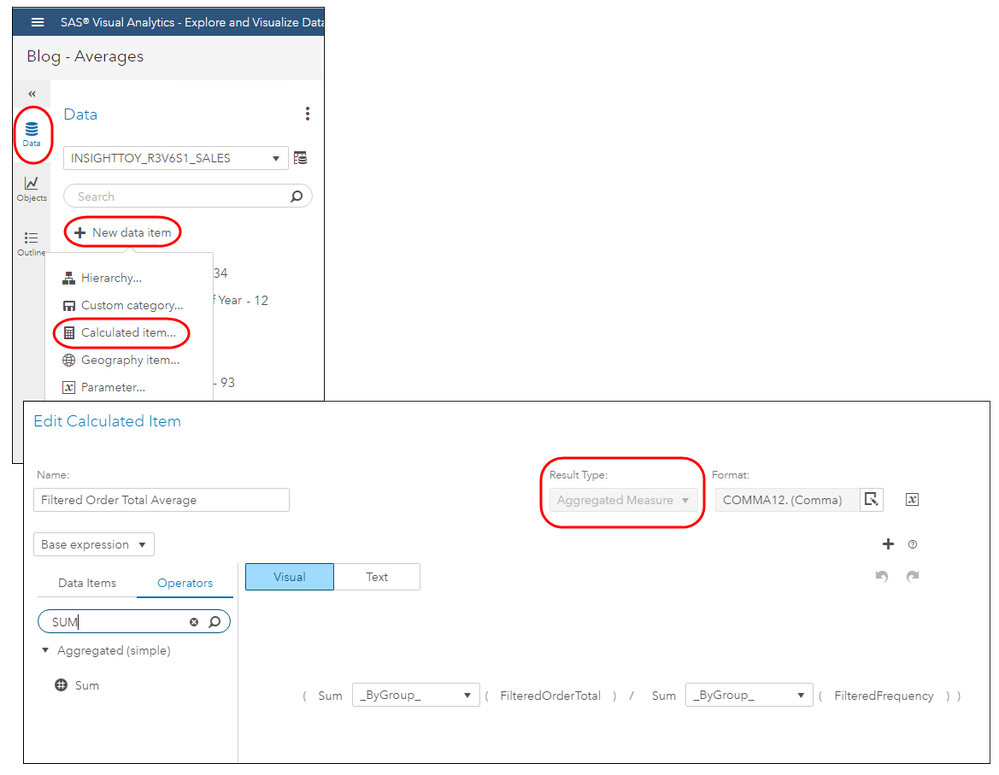## Conclusion

Now we have our total data's average available to compare with the selected month's average. We can use this in an object of our choice, such as a Bar Chart.   As a development tip, a nice way to check to be sure your expressions are returning the expected results, use a List Table. In the end, you could even define a Linked Selection Action between the two objects. That means when you select a value in one object the corresponding value is highlighted in the other object.Hi, I'm trying to recreate this in VA 7.3 by comparing a group average to a total average, but I can't get it to work. I have already calculated my by-group average in EG, and then derived my total average in VA. But I don't understand how the parameter selection doesn't also apply to the Order Total Average in your example?

When I add my by_group average and my total_average to a gauge, then make a selection from the parameter, it filters both values to the same amount.

Thank you!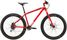Nice trick!

I will use this.

Possible improvement(?):

If you let the calculated item FilteredOrderTotal return missing in the else clause. Wouldn't that make it possible to get the average directly using avg()? And skip the frequency count?Hi Fredrik,

Yes - I just verified your improvement recommendation.  If you return Missing in the Else clause for FilteredOrderTotal then you can change the aggregation to Average and it returns the correct value.

Thanks for  the improvement recommendation.

TeriVersion history
Last update:
‎04-04-2018 06:26 PM
Updated by:Contributors
Article Labels
Article Tags